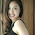## Sunday, December 9, 2012

### How to validate the special character on SharePoint List Column

Step 1: Open Column Settings for which column you want to add the validations:

List Settings > Change Column > Column Validation (below Column Settings)

Step 2: Add the following code at the Column Validation:

=AND(IF(ISERROR(FIND(",",[ColumnName])),TRUE),IF(ISERROR(FIND("&",[ColumnName])),TRUE),IF(ISERROR(FIND("!",[ColumnName])),TRUE),IF(ISERROR(FIND("@",[ColumnName])),TRUE),IF(ISERROR(FIND("~",[ColumnName])),TRUE),IF(ISERROR(FIND("#",[ColumnName])),TRUE),IF(ISERROR(FIND("\$",[ColumnName])),TRUE),IF(ISERROR(FIND("%",[ColumnName])),TRUE),IF(ISERROR(FIND("*",[ColumnName])),TRUE),IF(ISERROR(FIND("(",[ColumnName])),TRUE),IF(ISERROR(FIND(")",[ColumnName])),TRUE),IF(ISERROR(FIND("-",[ColumnName])),TRUE),IF(ISERROR(FIND("+",[ColumnName])),TRUE),IF(ISERROR(FIND(":",[ColumnName])),TRUE),IF(ISERROR(FIND(";",[ColumnName])),TRUE),IF(ISERROR(FIND("[",[ColumnName])),TRUE),IF(ISERROR(FIND("]",[ColumnName])),TRUE),IF(ISERROR(FIND(".",[ColumnName])),TRUE),IF(ISERROR(FIND("/",[ColumnName])),TRUE),IF(ISERROR(FIND("\",[ColumnName])),TRUE))

1.www.gofastek.com

2.Hi, any idea why this doesn't work for question marks?
Thanks

3.or in fact full stops. It doesn't kick up a validation error and instead goes straight to the error creating the list entry.

4.Thank you so much for this post. Special characters interfere with workflows and this looks like a viable solution.